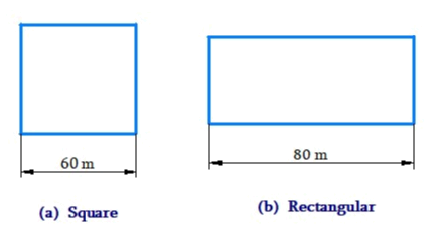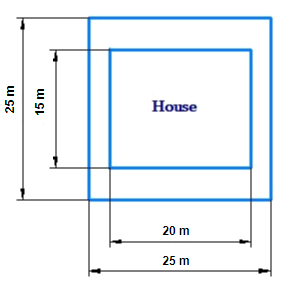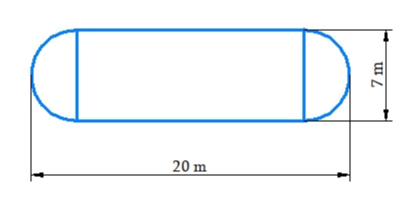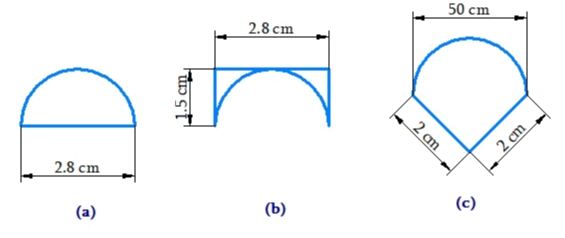NCERT Class 8 Maths Mensuration

# NCERT Class 8 Maths Mensuration

The chapter 11 begins with an introduction to Mensuration by recalling the basic concepts of perimeter and area of various plane figures such as triangles, rectangles, circles etc.Area of trapezium, area of a general quadrilateral and area of special quadrilateral are dealt in detail.After this, the area of a polygon is discussed in detail.After this, the surface area of cube, cuboid and cylinder is explained.Similarly, the volume of cube, cuboid and cylinder are dealt in the next section of the chapter.Finally , the volume and capacity is explained in the last section.

## Chapter 11 Ex.11.1 Question 1

A square and a rectangular field with measurements as given in the figure have the same perimeter. Which field has a larger area?

### Solution

What is Known?

Perimeter of rectangular field and square area same and also one side of square and rectangular field are known.

What is unknown?

Area of square and rectangular field.Reasoning:

It is given that perimeter of the square and the rectangular field are same, so we can find breadth of the rectangular field and also the area of the rectangular field.

Steps:

Side of the square $$= 60\rm\,m$$

Length of the rectangle $$= 80\rm\,m$$

Perimeter of the square \begin{align} &= 4 \times {\text{side of square}}\\ &= 4 \times 60 {\rm{m}} = 240 {\rm{m}} \end{align}

Perimeter of rectangle $$= 2 \times ({\rm{length}} + {\rm{breadth}})$$

Perimeter of square $$=$$ Perimeter of rectangle

\begin{align}240 &= \! 2\! \times \!( \! {\rm{length}} \!+\! {\rm{breadth}}\!)\\240 &= 2 \times (80 + {\rm{breadth}})\\240 &= 160 + 2 \times {\rm{breadth}}\\240 - 160 &= 2 \times {\rm{breadth}}\\{\rm{80 }}&= 2 \times {\rm{breadth}}\end{align}

${\rm{breadth}} = \frac{{80}}{2} = 40\rm\,m$

Area of the square

\begin{align} &= {\rm{side}} \times {\rm{side}} \\ &= 60 \times 60 \\ &= 3600{{\rm{m}}^2}\end{align}

Area of the rectangular field

\begin{align} &= {\rm{length}} \times {\rm{breadth}}\\ &= 80 \times 40 = 3200{{\rm{m}}^2}\end{align}

Thus, area of square is larger than the area of rectangular field.

## Chapter 11 Ex.11.1 Question 2

Mrs. Kaushik has a square plot with the measurement as shown in the figure. She wants to construct a house in the middle of the plot. A garden is developed around the house. Find the total cost of developing a garden around the house at the rate of $$55$$ per$${{\,\rm{m}}^2}$$.### Solution

What is Known?

The cost of developing a garden around the house at the rate of per $$\rm m^2$$ and also dimensions of the house and square plot.

What is unknown?

Total cost of developing of garden around the house.

Reasoning:

The plot is square from outside while the area of the house to be constructed is rectangular in the middle of the plot. From the diagram, we can see that the area of the garden is the difference between area of the square plot and the area of the house.

Steps:

Area of the square plot

\begin{align} & = {\rm{side}} \times {\rm{side}} \\ &= 25{\rm{m}} \times 25{\rm{m}} \\ &= 625{{\rm{m}}^2} \end{align}

Area of the house

\begin{align}&=\text{length}\times \text{breadth}\\ & =15\text{m}\times 20\text{m}\\ &=300 \; {{\text{m}}^{2}} \end{align}

Area of the garden to be developed $$=$$ (Area of the square plot ) $$-$$ (Area of the house)

\begin{align} &= 625{{\rm{m}}^2} - 300{{\rm{m}}^2}\\ &= 325{{\rm{m}}^2}\end{align}

Hence Area of the garden to be developed

$$= 325 \, \rm m^2$$

The cost of developing garden around the house

$$= {\rm{Rs}}.55\,\rm\,per\,{m^2}$$

$$\therefore$$ Total cost of developing a garden of area $$325\,{{\rm{m}}^2}$$

\begin{align} &= {\rm{Rs}}.325 \times 55 \\ &= {\rm{Rs}}.17,875\end{align}

## Chapter 11 Ex.11.1 Question 3

The shape of a garden is rectangular in the middle and semicircular at the ends as shown in the diagram. Find the area and the perimeter of this garden [Length of rectangle is $$20 – (3.5 + 3.5)$$ meters].### Solution

What is Known?

Shape of a garden is rectangular in the middle and semicircular at the ends.

What is unknown?

Area and perimeter of the garden

Reasoning:

The garden is rectangular in the middle and semicircular at the ends. From the diagram, we can see that the area of the garden is the sum of the rectangular middle portion and two semicircles at the end.

Steps:

Length of the rectangular part

\begin{align} &= 20-\left( {3.5 + 3.5} \right){\rm{ meters}}\\ &= \left( {20 - 7} \right){\rm\;{ meters}}\\ &= 13{\rm{ meters}}\end{align}

Breadth of the rectangular part $$=7$$ meter

Area of the rectangular part

\begin{align} &= {\rm{length}} \times {\rm{breadth}}\\ &= 13\,{\rm\;{meter}} \times 7\,{\rm{meter}}\\ &= 91{\rm{ m}}{\rm{. sq}}{\rm{.}}\end{align}

Diameter of the semi circle $$= 7 \rm\,m$$

Radius of the semi circle =\begin{align}\frac{7}{2}\end{align}m $$= 3.5 \rm\,m$$

Area of the semi circle \begin{align} = \frac{1}{2} \times \pi \times {r^2}\end{align}

Area of two semi circles

\begin{align} &= 2 \times \frac{1}{2} \times \frac{{22}}{7} \times {(3.5)^2}\\&= \frac{{22}}{7} \times 12.25\\ &= 38.5\,\,{{\rm{m}}^2}\end{align}

Total area of the garden = (Area of rectangle) + (Area of two semicircle regions)

\begin{align}& = 91{{\rm{m}}^2} + 38.5{{\rm{m}}^2}\\ &= 129.5{{\rm{m}}^2}\end{align}

Perimeter of the garden = length of rectangle + perimeter of two semicircle + length of rectangle

\begin{align}&= \,13 + 2\left( {\pi \times 3.5} \right) + 13\\&= \,26 + 7\pi \\&= \,26 + \not\!{7}\times \frac{{22}}{{\not\!{7}}}{\rm{m}}\\&= \,26 + 24{\rm{m}}\\&= \,48{\rm\;{m}}\end{align}

## Chapter 11 Ex.11.1 Question 4

A flooring tile has the shape of a parallelogram whose base is $$24 \rm\,cm$$ and the corresponding height is $$10 \rm\,cm.$$ How many such tiles are required to cover a floor of area $${\rm{1080}}\,\,{{\rm{m}}^2}$$? (If required you can split the tiles in whatever way you want to fill up the corners).

### Solution

What is Known?

Shape of the tile and its dimensions.

What is unknown?

Number of tiles that are required to cover a floor of area$$=$$$$1080\,\,{{\rm{m}}^2}$$

Reasoning:

Shape of the tile is parallelogram and its area can be found easily.

Area of parallelogram $$=$$ Base $$\times$$ Height

Steps:

Area of parallelogram

\begin{align} &= \text{base}\times \text{height} \\ &=24\,\text{cm}\times 10\,\text{cm}\\&=240\,\text{c}{{\text{m}}^{2}}\end{align}

Hence the area of one tile $$= 240\,{\rm{c}}{{\rm{m}}^2}$$

Area of the floor $$= 1080{{\rm{m}}^2}\,\,\left( {{\rm{given}}} \right)$$
$$\therefore$$ Required number of tiles \begin{align} = \frac{{{\text{Area of the floor}}}}{{{\text{Area of one tile}}}}\end{align}

\begin{align}& =\frac{1080\,{{\text{m}}^{2}}}{240\,\,\text{c}{{\text{m}}^{2}}} \\ & =\frac{(1080\!\times \!10000){{\text{cm}}^{2}}}{240\text{c}{{\text{m}}^{2}}}\\&(\because 1\,\text{m }\!\!=\!100\,\text{cm}) \\ & =45000\,\,\,\text{tiles} \\\end{align}

Thus,$$45000$$ tiles are required to cover a floor of area $$1080 \rm{m^2}$$

## Chapter 11 Ex.11.1 Question 5

An ant is moving around a few food pieces of different shapes scattered on the floor. For which food-piece would the ant have to take a longer round? Remember, circumference of a circle can be obtained by using the expression $$c = 2{\rm{\pi }}r$$ , where $$r$$ is the radius of the circle.### Solution

What is Known?

Shape and dimensions of various food pieces.

What is unknown?

Perimeter of different shaped food pieces.

Reasoning:

Hence, we will calculate perimeter of different shaped food piece. Larger the perimeter, longer will be the path to take round.

Steps:

(a) Radius of the semicircle part

\begin{align} = \left( {\frac{{2.8}}{2}} \right)\,{\rm{cm}} = 1.4\,{\rm{cm}}\end{align}

Perimeter of the circle $$= 2{{ \pi }}r$$

$$\therefore$$ Perimeter of the semicircle = $$\pi r$$

The perimeter of the food piece

\begin{align}&= 2.8\,{\rm{cm}} + \pi r\\ &= 2.8\,{\rm{cm}} + \left( {\frac{{22}}{{\not7}} \times{{\not\!\!\!\!{1.4}}^{0.2}}} \right)\,{\rm{cm}}\\ &= {\rm{ }}2.8\,{\rm{cm }} + {\rm{ }}4.4\,{\rm{cm}}\\ &= 7.2\,{\rm{cm}}\end{align}

\begin{align} = \frac{{2.8}}{2} = 1.4\,\,{\rm{cm}}\end{align}

Perimeter of the food piece

\begin{align}&= 1.5\,{\rm{cm}} + 2.8\,{\rm{cm}} + 1.5\,{\rm{cm}} + \pi r\\&= 5.8\,{\rm{cm}} + \left( {\frac{{22}}{{\not7}} \times \not\!\!\!\!{{1.4}^{0.2}}} \right){\rm{cm}}\\&= 5.8\,\,{\rm{cm}} + 4.4\,\,c{\rm{m}}\\& = 10.2\,\,{\rm{cm}}\end{align}

(c). Radius of the food piece

\begin{align} = \frac{{2.8}}{2} = 1.4\,\,{\rm{cm}}\end{align}

Perimeter of the food piece

\begin{align}&= 2{\rm{cm}} + \pi r + 2{\rm{cm}}\\& = 4\,{\rm{cm}} + \left( {\frac{{22}}{{\not7}} \times \not\!\!\!\!{{1.4}^{0.2}}} \right){\rm{cm}}\\& = 4\,\,{\rm{cm}} + 4.4\,\,c{\rm{m}}\\& = {\rm{8}}{\rm{.4}}\,\,{\rm{cm}}\end{align}

Thus, the ant will have to take a longer round for food piece (b) because the perimeter of the figure given in (b) is the greatest among all.

Mensuration | NCERT Solutions
Instant doubt clearing with Cuemath Advanced Math Program